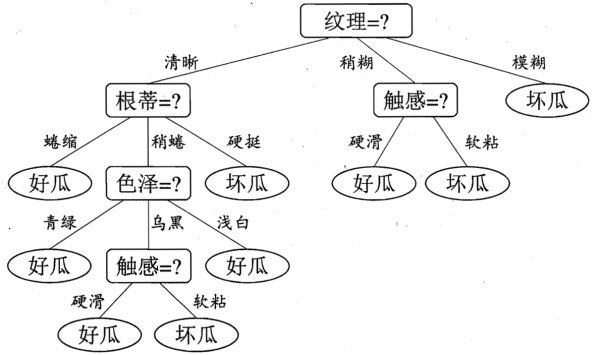# 决策树• 算法比较简单；
• 理论易于理解；
• 对噪声数据有很好的健壮性。

## 划分选择

$$Ent(D)=-\sum^y_{k=1}p_klog_2p_k$$

$$Gain(D, a) = Ent(D) - \sum^V_{v=1} \frac{|D^v|}{D} Ent(D^v)$$

## 常用算法

• ID3算法：以信息增益为准选择决策树的属性，注意信息增益偏好可取值数目较多的属性。
• C4.5算法：使用增益率选择最优划分属性，增益率定义为 $$Gain_ratio(D, a) = \frac{Gain(D,a)}{IV(a)}$$，而 $$IV(a) = - \sum^V_{v=1}\frac{|D^v|}{D} log_2\frac{|D^v|}{D}$$。注意增益率偏好可取值数目较少的属性。
• 基尼指数：这是CART决策树使用的划分算法，$$Gini_{index}= \sum^V_{v=1} \frac{|D^v|}{D} Gini(D^v)$$ ，而 $$Gini(D)=-\sum^y_{k=1} \sum_{k’!=k} p_kp_{k’} = 1- \sum^y_{k=1} p_k^2$$。

## 剪枝处理

• 预剪枝：在构造决策树的同时进行剪枝，对每个节点进行划分前的估计，如果不能带来决策树泛化性能的提升则停止划分并将当前节点标记为叶节点。预剪枝有带来欠拟合的风险。
• 后剪枝：决策树构造完成后进行剪枝，自底向上对非叶节点考察，如果该节点的子树替换为子树的叶节点可以提升泛化性能，则替换该子树为其叶节点。后剪纸的欠拟合风险很小，泛化性能通常优于预剪枝，但计算量大，训练时间较长。

## 连续与缺失值处理

• 连续值：连续属性离散化，比如二分法。
• 缺失值：让同一个样本以不同概率划入到不同的子节点中去。